472kHz.org

### Weak signal modes

#### Extreme narrow bandwidth modes

A simple and straightforward method to improve SNR is to reduce the signal bandwidth. As mentioned before the minimal receiver bandwidth for undistorted reception is determined by spectrum of the transmitted signal. In case of CW it is the (keying) speed that puts a limit to the minimal bandwidth. Thus reducing bandwidth means slowing down the keying speed.
An accepted method to measure the speed of CW is the PARIS system. The word Paris has a length of exactly 50 'dots', word spacing included. Based on this system a CW signal of 12 words per minute (WPM) means 600 'dot lengths' per minute or 10 'dot lengths' per second. But as each dot is separated by space of the same length the actual length of the 'dot cycle' is the double. If a continuous series of dots is given at 12 WPM this results in a 5 Hz square wave. If an RF signal is keyed with this series of dots you will create some kind of AM signal modulated with a 5 Hz 'tone', resulting in a carrier with 2 sidebands at 5 Hz. The useful bandwidth of this signal will be 10 Hz. Depending on how 'hard' the keying is, more sidebands further away from the carrier will be created but these do not contain additional information and can be considered a waste of energy (and a source of QRM to others). So basically the minimum bandwidth that is required for undistorted reception a CW signal is:Assuming that the only noise source is a frequency independent (white) noise, the total receiver noise will be directly proportional to the receiver bandwidth. Taking a 12 WPM CW signal as reference and assuming that the receiver bandwidth is optimised to the transmission speed the table below shows the SNR improvement that can be achieved by reducing the CW speed::

Speed Optimum bandwidth SNR vs 12 WPM
12 WPM 10 Hz
6 WPM 5 Hz 3 dB
1 sec./dot 1 Hz 10 dB
3 sec./dot 0.333 Hz 14.8 dB
10 sec./dot 0.1 Hz 20 dB
30 sec./dot 0.0333 Hz 24.8 dB
60 sec./dot 0.0167 Hz 27.8 dB
120 sec./dot 0.00833 Hz 30.8 dB

It is clear that a significant SNR improvement can be achieved by reducing the CW speed. As you can see from the table, for QRSS the speed is given in seconds per dot rather than WPM. At these very slow CW speeds it becomes rather difficult to copy the signal by ear as you would almost need a chronometer to time the dots and dashes. Another problem is that filters become more and more complicated to built as the bandwidth becomes smaller. And also tuning into a signal can be a rather tricky thing at bandwidths below 1 Hz.
So reducing bandwidth not only has benefits in the way of an improved SNR but creates also a lot of additional problems. The way to overcome these problems is the use of Digital Signal Processing (DSP).

Digital Signal Processing
Digital Signal Processing is one of these magic expressions that you hear once in a while, but seems the domain of specialized engineers and maybe some 'happy few' hams. In the early days special (and rather expensive) hardware was needed to perform DSP. But now all the special hardware can be replaced by a computer with sound card and the software you need is available for free. As the expression Digital Signal Processing says, the analog (input) signal is converted to digital, then processed and eventually converted back to an analog (output) signal or displayed in some way.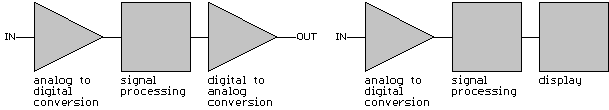The conversion of the analog signal to a digital form is done by analog-to-digital conversion (ADC). The most basic version of ADC is often done by ourselves when we use a voltmeter to determine the value of a voltage. With DSP this 'reading of voltages' is done automatically at a known time interval, this is called sampling. The result is a series of measurements, where we know the measured voltage and the time when it was measured.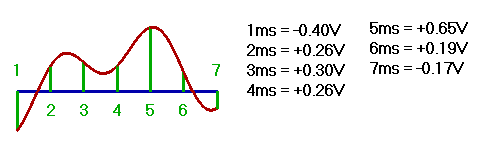These data are processed digitally, what in practice means that they undergo a series of more or less complicated calculations. The result can be interpreted as digital data or eventually converted back to an analog signal. Using DSP all kind of things can be done, not only filtering but also reducing bandwidth, time multiplexing of several signals etc...
Here we will only discuss the filtering of a signal. Although there are several methods to filter a signal digitally the major technique used for the reception of extreme narrow bandwidth modes is Fast Fourier Transform (FFT).

Fast Fourier Transform (FFT)
The mathematical background on this transform was developed by the French mathematician and physicist Joseph Fourier, about 200 years ago. The basic idea behind the Fourier transform is that any signal can be seen as the sum of a series of sinusoidal signals, where each sine can have a different amplitude and phase.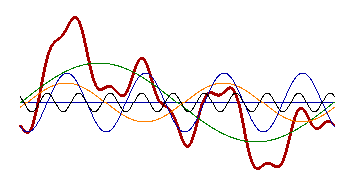In the above picture the complex red signal is equal to the sum of the green, blue, orange and black sines. The mathematical equations of the Fourier transform are rather complicated, those who are interested can have a look at:

Fortunately it is not necessary to go deep into these mathematics to understand how FFT works and therefore math will be kept to a minimum. But as there is a lot of calculating involved Fourier transforms will take a lot of 'computing time'. To reduce this a special algorithm was developed to enhance the speed of the Fourier transforms, this algorithm is called the Fast Fourier Transform (FFT).
When we take the Fourier transform of any signal we actually split the signal up in a number of sines and for each of these sines the amplitude and phase is calculated. Each of these sines represents a certain frequency (or better frequency band) and from these sum of sines (and their amplitudes) we can reconstruct the frequency spectrum of the measured signal.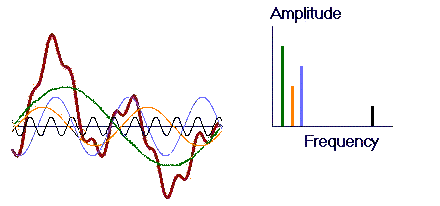The 'quality' of the reconstructed frequency spectrum depends on:

• The sample rate (interval between 2 A-D conversions)
• The sampling time for one transform
• The number of bits of the A-D converter

The sample rate determines the maximum frequency of the spectrum : the maximum frequency that can be reconstructed is 50% of the sampling frequency.
eg. : If we take a sample every 0.2 ms (equals a sampling frequency of 5 kHz) the maximum frequency that can be reconstructed is 2.5 kHz.
The sampling time determines the frequency resolution (or the bandwidth of each 'channel') : the frequency resolution is equal to one over the sampling time.
eg. : If we take a sampling time of 0.1 seconds the frequency resolution (or channel bandwidth) will be 10 Hz, this means that in the series of sines of the Fourier transform each sine will represent a 10 Hz wide channel.
The number of samples in a fast Fourier transform has to be a power of 2 (2, 4, 8, 16, ... , 256, ... 65536, ...). Although you can take any number of samples and just add a series of 'zeros' until you get a power of 2 it is more practical to choose the correct ratio between sample rate and sampling time in order to get the right number of samples.
eg. : if we have a sample rate of 0.2ms we will not take a sampling time of 0.1 seconds, what would result in 500 samples, but a sample time of 0.1024 seconds in order to get 512 samples (= 29). The result of the Fourier transform will be a series of 256 sines, each representing a 9.766 Hz wide channel between 0 Hz and 2.5 kHz.
The picture below shows a simple example, the Fourier transform of 16 samples at a rate of 1 ms results in a series of 8 sines that each represent a 62.5 Hz wide channel between 0 and 500 Hz:The number of bits of the A-D converter determines the dynamic range of the spectrum. For a sound card we can choose between  8 bit, 16 and sometimes even 24 bit A-D conversions.
eg. : For a 8-bit A-D conversion we have 28 = 256 levels and the dynamic range will be 20·Log(256) = 48 dB. For a 16-bit A-D conversion we have 216 = 65536 levels and the dynamic range will be 20·Log(65536) = 96 dB.

In CW the code QRS means 'send more slowly', hence QRSS was adopted as the name for extreme slow speed CW. To take advantage of the very narrow bandwidth of the transmitted signal an appropriate filter at the receiver end is needed. Making a 'software filter' using FFT has some advantages over the old fashioned hardware filter. One of the main advantages, when using it for reception of QRSS signals, is that FFT does not give you one single filter but you get a series of filters with which you can monitor a complete spectrum at once. This means that you do not have to tune exactly into the signal, what can be very delicate at sub-Hertz bandwidths. Also it is possible to monitor more than one QRSS signal at the same time. At first glance it looks as if it is complicated to do this, even if FFT presents you this nice multi-channel filter it might seem difficult to monitor all these channels. Further the long duration of the dots and dashes is unfavorable for aural monitoring.
A solution to the above problems is to show the outcome of the FFT on screen rather than making it audible. The result is a graph where one axis represents time, the other axis represents frequency and the colour represents the signal strength. If the vertical axis represents time we call it a waterfall display while it is called a curtain display if the horizontal axis represents time.
All this may sound complicated but it is easy to understand when you see an example (curtain display):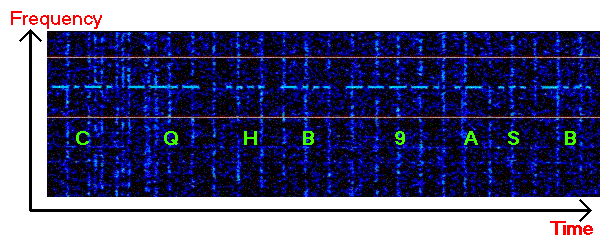The picture above shows the signal of HB9ASB that was not audible due to very strong QRN, the vertical lines are the result of S9++ static crashes.
Some nice collections of screen captures can be found at the web pages of DK8KW, OK1FIG and NL9222.

Dual Frequency CW (DFCW)
At a speed of 3 seconds per dot a very basic QRSS QSO will take about 30 minutes. Changing QRN levels and/or propagation during this period can have a vast effect on a QSO. Therefore a new transmission mode has been developed that enhances the average speed by a factor of 2.5 to 3.
When the nature of CW is analysed it seems a digital mode where 'key down' represents a logic '1' and 'key up' a logic '0'. But another approach it is to see it as a mode with 3 'logical states' : the 'dash' (3 periods of key down + 1 period of key up or '1110'), the 'dot' (1 period of key down + 1 period of key up or '10') and the 'character space' (2 periods of key up or '00'). The spacing between words is 3 character spaces. So there are 2 elements that play a role : the presence/absence of a signal and the duration of the signal. As CW was intended to be received by ear the different duration of the signals is essential, but it lengthens the time needed to transmit a text.
In Dual Frequency CW (DFCW) the element 'duration' is replaced by the element 'frequency'. So dots and dashes no longer have a different length but they are transmitted on a different frequency. Due to this frequency shift there is no 'space' needed between the dots / dashes and the character space can be reduced to the same (dot)length.
When the idea of DFCW first was introduced there was a lot of skepticism about the readability of there frequency shifted signals but in practice it seems rather easy to read it from the screen. To make it even more easy to read, especially during a sequence of dots or dashes, a short space (typically 1/3 of a dot length) is added between the dots and dashes. This reduces the average speed a bit, but is improves the readability and also reduces the duty cycle (what is better for the PA).
The example below shows the text 'CQ ON7YD K' in QRSS and DFCW, at the same speed :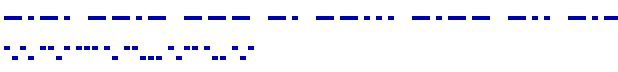At a speed of 3 seconds per dot this CQ will take 5'30" in QRSS while it will take only 1'54" in DFCW. The speed advantage of DFCW over QRSS can be taken in 2 ways, either by reducing the duration of a QSO or by increasing the dot length and working at a narrower bandwidth. The last means that, for the same duration of a QSO, the dot length in DFCW can be 2.5 to 3 times longer and as a result of this get a 4 to 5 dB better SNR.
The screen shot below shows a 'real-world' picture of a DFCW signal. This should prove how easy it is to decode a DFCW signal by eye.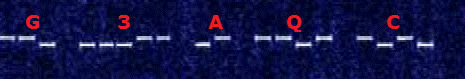Dot length and SNR
In theory a QRSS signal with 3 seconds dot length should have a almost 15 dB better SNR than a 12 WPM CW signal. And if you are not in a hurry, increasing the dot length to 2 minutes should outperform a 12 WPM CW signal by over 30 dB. But what about reality? Below you find some real world test as done by DL8KW, W1TAG, G3YXM and G3NYK.

In April 2000 Geri Kinzel (DK8KW) did some measurements to compare QRSS with normal (aural) CW:
This morning I made some laboratory tests to get some indication about the ability to communicate with signals below noise level using QRSS. I used a calibrated frequency synthesiser (Adret 2230), an 0-120 dB attenuator in 1 dB steps (Schlumberger BMD500) and my Praecitronic MV61 Selective Level Meter. With a BNC T-connector I fed the normal band noise including LORAN lines on 137.500 kHz (+/- 50 Hz) to one side and the output of the frequency synthesiser to the other side. With the attenuator I made sure that a 0 dBm (50 Ω reference) signal with the synthesiser corresponds to a -80 dBµ ( 0 dBµ = 0.775 V into 75 Ω = +9 dBm, -80 dBµ = -71 dBm) signal at the MV62 (plus/minus 1 dB). The band was quite, with a background noise around -110 dBµ (S4, -101 dBm) and LORAN lines clearly visible. Using the 100 Hz bandwidth of the MV62 and the cascaded 250 Hz/500 Hz CW filters of the IC-746 I checked the signal by ear as well as with the Spectrogram software with the normal parameters I use for '3-5 second dot length' QRSS (5.5k sample rate, 16 bit mono, 16384 points FFT = 0.3 Hz resolution, 60 dB scale, 300 ms time scale, 10 x average) and obtained the following results:

Signal strength at RX input
Comment
-100dBµ / -91dBm
good audible CW (S6)
-110dBµ / -101dBm
CW signal equal to noise level (S4), can just be copied
-115dBµ / -106dBm
boundary for aural CW, signal just detectable by ear
-125dBµ / -116dBm
-130dBµ / -121dBm
-135dBµ / -126dBm
just detectable QRSS signal ('T' report)
-140dBµ / -131dBm
signal not detectable

Conclusions:
QRSS has a 20 dB signal level advantage over normal (aural CW), which means that the minimum detectable and/or readable QRSS signal that might just allow communication lies 20 dB below the signal, that can just be detected and/or decoded by a trained CW-operator's ear. If I consider the "CW-operator's ear/brain bandwidth" to be 30 Hz, this roughly corresponds to the bandwidths used (0.3 versus 30 Hz).

John Andrews (W1TAG) did also some measurements. He was using an Icom R75 receiver and 6 foot loop to receive the signal transmitted by a 10 mW exciter, feeding a small loop antenna through a variable attenuator. The receiver was picking up whatever noise was present at the time of the test. The signal was decoded using the ARGO software.
The transmitted signal was attenuated to a level that just allowed a solid copy:

Dot length Screenshot Measured level
0.2 sec
(6 WPM)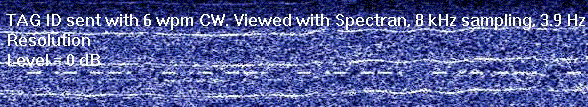reference
3 sec.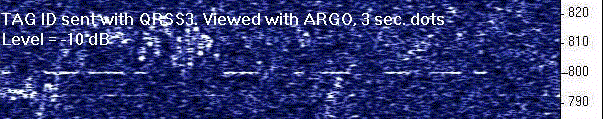-10 dB
10 sec.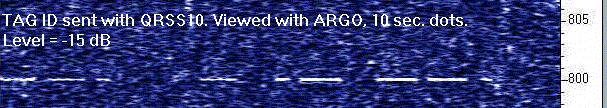-15 dB
30 sec.-19 dB
60 sec.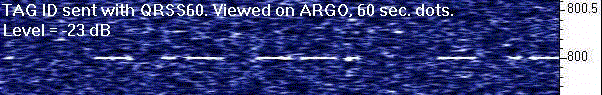-23 dB

For all measurements there is a ± 2 dB difference between the measured and theoretical result, but I believe that this can be explained by the fact that the 6 WPM reference screen shot has a clearly lower SNR than the other screen shots. The full report on this measurement can be found here.

In March 2002 Dave Pick, G3YXM, and Alan Melia, G3NYK, compared normal CW and QRSS at speeds of 3 sec/dot, 10 sec/dot and 60 sec/dot over a 220 km path. G3YXM was transmitting and reduced power until the signal just reached the 'O copy' level at G3NYK :

Dot length Antenna power Power versus 12 WPM
0.1 sec. (12 WPM) 360 mW reference
3 sec. 23 mW -12 dB
10 sec. 3.9 mW -20 dB
60 sec. 0.6 mW -28 dB

The full report of this test can be found here.
Dave states that he needs to run 2 kW RF into his antenna to achieve 1 W ERP. This means that the ERP at the 60 sec/dot test was no more than 300 nW (yes, nano-Watt), not bad at all to cover a distance of 220 km.

For digital modes the sensitity or threshold level of often declared at SNR at 2.5 kHz bandwidth. As the threshold level depends on several factors, including the operator, it is difficult to give an exact value, but an experienced operator should be able to copy signals down to a SNR of -26 dB.

It is clear that increasing the dot length will improve SNR. But there are limits to the maximum usable dot length:

• TX and RX stability: the combined drift of transmitter and receiver must less that the signal bandwidth during the length of 1 dash. For 3 seconds dot length this means a maximum combined drift of 0.333 Hz over a 9 second period (or less than 2.22 Hz/minute). For 120 seconds dot length the combined drift must be less than 0.00833 Hz over a 2 minute period (or less than 0.0416 Hz/minute).
• Ionospheric effects: communication over long distances involves almost always ionospheric propagation. The ionospheric layers are however not stable, they change intensity and move around all the time. This causes a Doppler effect on the signal. It is obvious that it will have a negative effect on the SNR if this frequency shift exceeds the signal bandwidth.
• Propagation: a QRSS QSO, or even just transmitting a callsign, will take a lot of time. Time needed for a basic QRSS QSO varies from about 30 minutes (3 seconds dot length) to over 10 hours (120 seconds dot length). Propagation must allow such QSO lengths.

When monitoring QRSS signals one might observe a strange effect on very strong signals. The dots and dashes are widened at the begin and end, producing so called 'bones'. This can be seen in the picture below (signal of PA0BWL copied by NL9222):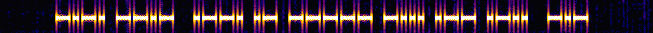In order to obtain optimum SNR the FFT length must be more or less equal to the dot length. But that means that the FFT should begin at the same time as the dot, any time shift between the keying of the QRSS signal and the FFT's will cause line in between the dots. If the time shift between a series of dots and the FFT's is 50% of dot length this will even produce a uniform line on the screen with no dots visible anymore: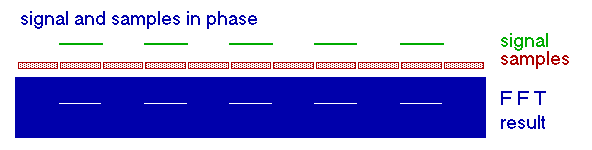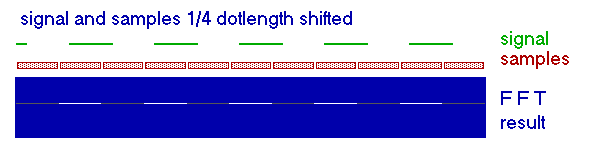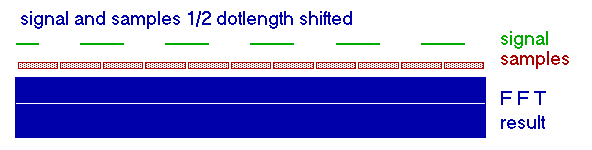To avoid this effect the keying at the TX end and FFT at the RX end have to be synchronised, what often is not possible. Fortunately there is a simple solution to this problem. Instead of using a complete new set of samples for each FFT, only partly new data taken and the existing data is shifted up in the data-array used to perform the FFT: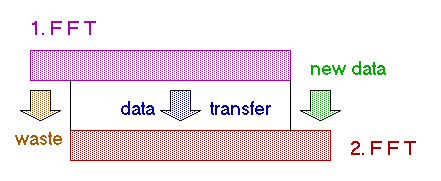This method has the advantage that the length of the FFT array can be equal to the dot length, but there are also some disadvantages. First of all the workload for the computer increases significantly, as much more FFT's are required. And this technique produces 'bones' with strong signals.

Another way to improve the SNR is averaging. It is based on the fact that noise is random and cancels itself out over a number of measurements while the signal is consistent. Therefore the results of several FFT's are added and the average is taken. While the advantage is an improved SNR the disadvantage is that the results on screen appear slower, as you have only 1 output to screen for several FFT's. Fortunately there is a way around this, but it has also some drawbacks. Alberto di Bene (I2PHD), one of the programmers of Spectran, sent me some interesting comments on how averaging can be done:
In Spectran there are two averaging mechanisms :

• A) the averaging that you can activate with the AVG button on the main panel. This has two sub-choices : the sliding window and the integration radio buttons. With the sliding window a moving average is performed on the computed spectrum, and a result is output for each input spectrum, no matter what the averaging factor is. This factor has only the effect of adjusting the width of the moving window. On the contrary, when doing integration, if the the averaging factor is N, an output spectrum is generated every N input spectra, simply taking their average.
• B) the averaging which is produced as a side effect of the overlapping done on the input time series. The purpose of this overlapping is to produce an output in a time shorter of what would otherwise be needed, should overlapping not be used. To clarify a little : suppose that the sampling rate is 8000 Hz, and the resolution set to 0.12207 (rounded in the display to 0.12) Hz. This implies that the Nyquist frequency is 4000 Hz, and you have 4000 / 0.12207 magnitude values, obtained with 8000 / 0.12207 complex values (the real and the imaginary parts). So you have to compute 8000 / 0.12207 = 65536 complex values, corresponding to an equal amount of real time samples. The time needed for this is 65536 / 8000 (the number of samples you get each second) = 8.192 seconds. And, actually, if you set Spectran with the speed slider at its minimum (the slider colour changes to green), i.e. an overlapping factor of 1, you will have a refresh of the screen about every 8 seconds. Things go worse with increasing resolutions, the wait time increases proportionally. If a faster refresh rate is desired, the overlapping can be of help. With this method, you don't wait that a complete new set of input values is ready, but a part of the old data is reused, together with some new ones. Of course there is a compromise (no free lunch...) : in reusing the old data, you loose some time resolution, and this appears on the screen as a smearing, or blurring, of the spectral lines. This effect increases with the overlapping factor. With factors up to about 8, it is not very noticeable, but things go worse with higher values. It's the price to be paid for having a quicker refresh rate. As with almost any choice in life, a suitable compromise must be found, and this has also implications on the optimal dot/dash length.

Operating practice
Operating QRSS and DFCW is rather simple, just a few 'specialities':

• You need a stable TX: long term stability of 5 Hz is an absolute minimum, 1 Hz or better is recommended (for dot lengths in excess of 10 seconds stability should at least 1 Hz, recommended 0.1 Hz or better).
• Keep your CQ's short: eg. CQ G3XXX K not CQ CQ CQ G3XXX G3XXX G3XXX PSE K.
• The report system is the TMO system (similar to EME):
• T = signal traces seen but not good enough for a QSO.
• M = weak signal but good enough for a QSO.
• O = perfect copy.
• For intra-continental QSO's (eg. within Europe):
• A dot length of 3 seconds is recommended, eventually 10 seconds if weak signals are expected
• For DFCW a shift of 2 to 5 Hz (with 'dash' being the higher frequency) and a key gap of about 1/3 of the dot length is recommended
• For inter-continental (DX) tests:
• Dot lengths up to 120 seconds are common.
• For DFCW a shift of 0.1 to 0.5 Hz (with 'dash' being the higher frequency) is recommended.
• During a QSO (once you are sure that both stations have the calls OK) you can use the suffixes instead of complete calls.
• If you see the end of a QSO and you want to contact one of the stations you can start calling this station while the previous QSO is going on, just call on another frequency.
• If replying to a CQ it is recommended to call NOT exactly on the frequency of the other station, this will avoid QRM in case more than 1 station is responding.
• Due to the slow 'transmission rate' it is recommended to limit the QSO to exchange of callsigns and reports, especially if you are located close to another radioamateur that is active in this mode (one strong signal can 'block' the entire QRSS segment).

A basic QRSS (or DFCW) QSO could look like this:

• [ON7YD transmitting] CQ ON7YD K
• [G3XDV transmitting] ON7YD G3XDV K
• [ON7YD transmitting] G3XDV YD OOO K
• [G3XDV transmitting] YD XDV OOO K
• [ON7YD transmitting] XDV YD TU 73 K
• [G3XDV transmitting] YD XDV GL 73 SK

At very slow speeds (dot lengths of 30 seconds and more) it is advised to keep the exchanged information even shorter, in order to keep the duration of a QSO within reasonable limits:

• [G3AQC transmitting] CQ G3AQC K
• [VA3LK transmitting] G3AQC VA3LK K
• [G3AQC transmitting] VA3LK AQC OO K
• [VA3LK transmitting] AQC LK OO K
• [G3AQC transmitting] LK AQC SK

Crunch: a completely different approach to decode QRSS signals
A completely different approach to 'decode' QRSS transmissions was made by Bill de Carle, VE2IQ. With Crunch the incoming audio signal is recorded as a WAV-file, either using the soundcard or VE2IQ's Sigma-Delta DSP board. Afterward the file is filtered and 'speeded up' to bring the QRSS signal to normal speed CW, audible via the soundcard.
The DSP software performs the following steps:

1.Optionally: pass the incoming audio through a narrow bandpass filter centered on 800 Hz. This is a good idea when receiving slow-speed CW because the signal bandwidth is minimal. The filter has a Butterworth shape and a width (at the -3 dB points) of 50 Hz.
2. Mix the signal down to a target frequency of 22.5 Hz.
3. Lowpass the output from the above mixer stage, then reduce the sampling rate by some 32 times. Since there are no high frequency components left at this point, we can completely capture the processed audio tone with 225 samples per second, well over the Nyquist rate.Save the new sampled waveform into a 16-bit PCM (.wav) file, stating in the .wav header the sample rate was 8000 samples per second.

Saying that the sample rate was 8000 samples per second where in fact it was only 225 samples per second results in a factor 35.6 time compression (8000/225) and multiplies thus the nominal 22.5 Hz derived carrier frequency back up to 800 Hz, where it can easily be heard with the human ear.

 previous content next